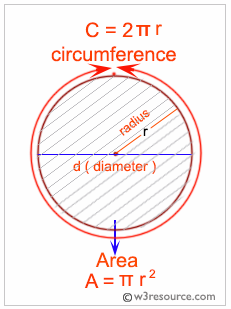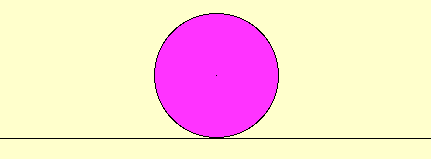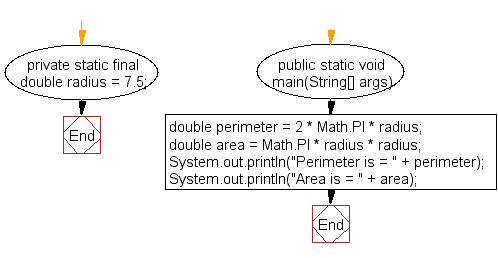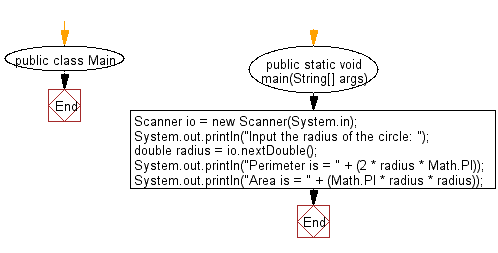﻿ Java exercises: Find the area and perimeter of a circle - w3resource# Java Exercises: Find the area and perimeter of a circle

## Java Basic: Exercise-11 with Solution

Write a Java program to print the area and perimeter of a circle.

In geometry, the area enclosed by a circle of radius r is πr2. Here the Greek letter π represents a constant, approximately equal to 3.14159, which is equal to the ratio of the circumference of any circle to its diameter.

The circumference of a circle is the linear distance around its edge.

Pictorial Presentation:Why is the area of a circle of a circle pi times the square of the radius?Sample Solution:

Java Code:

``````
public class Exercise11 {

private static final double radius = 7.5;

public static void main(String[] args) {

double perimeter = 2 * Math.PI * radius;

System.out.println("Perimeter is = " + perimeter);
System.out.println("Area is = " + area);
}
}
```
```

Sample Output:

```Perimeter is = 47.12388980384689
Area is = 176.71458676442586
```

Flowchart:Sample Solution:

Java Code:

``````import java.util.Scanner;
public class Main {
public static void main(String[] args) {
Scanner io = new Scanner(System.in);
System.out.println("Input the radius of the circle: ");
System.out.println("Perimeter is = " + (2 * radius * Math.PI));
}
}
```
```

Sample Output:

```Input the radius of the circle:
5
Perimeter is = 31.41592653589793
Area is = 78.53981633974483
```

Flowchart:Java Code Editor:

What is the difficulty level of this exercise?

Test your Programming skills with w3resource's quiz.

﻿

## Java: Tips of the Day

countOccurrences

Counts the occurrences of a value in an array.

Use Arrays.stream().filter().count() to count total number of values that equals the specified value.

```public static long countOccurrences(int[] numbers, int value) {
return Arrays.stream(numbers)
.filter(number -> number == value)
.count();
}
```

Ref: https://bit.ly/3kCAgLb